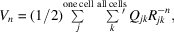International
Tables for
Crystallography
Volume B
Reciprocal space
Edited by U. Shmueli

International Tables for Crystallography (2010). Vol. B, ch. 3.4, p. 449   | 1 | 2 |

## Section 3.4.2. Definition and behaviour of the direct-space sum

D. E. Williamsa

aDepartment of Chemistry, University of Louisville, Louisville, Kentucky 40292, USA

### 3.4.2. Definition and behaviour of the direct-space sum

| top | pdf |

This pairwise sum is taken between atoms (or sites) in the reference unit cell and all other atoms (or sites) in the crystal, excluding the self terms. Thus, the second atom (or site) is taken to range over the entire crystal, with elimination of self-energy terms. Ifrepresents an energy, each atom is assigned one half of the pair energy. Therefore, the energy per unit cell iswhereis a given coefficient,is an interatomic distance, and the prime on the second sum indicates that self terms are omitted. In the case of the Coulombic sum,andis the product of the site charges.

Table 3.4.2.1gives an example of the convergence behaviour of the untreatedCoulombic sum for sodium chloride. Even at the rather large summation limit of 20 Å the Coulombic lattice sum has not converged and is incorrect by about 8%. The 20 Å sum included 832 molecules and 2494 individual distances. At various smaller summation limits the truncation error fluctuates wildly and can be either positive or negative. Note that the results shown in the table always refer to summation over whole molecules, that is, over neutral charge units.

 Table 3.4.2.1| top | pdf | Untreated lattice-sum results for the Coulombic energy (n = 1) of sodium chloride (kJ mol−1, Å); the lattice constant is taken as 5.628 Å
Truncation limitNumber of moleculesNumber of termsCalculated energy
6.0 23 67 −696.933
8.0 59 175 −597.371
10.0 108 322 −915.152
12.0 201 601 −773.475
14.0 277 829 −796.248
16.0 426 1276 −826.502
18.0 587 1759 −658.995
20.0 832 2494 −794.619
Converged value −862.825

If the Coulombic summation is not carried out over neutral charge units the truncation error is even larger. These considerations support the conclusion that accelerated-convergence treatment of the Coulombic lattice sum should be regarded as mandatory. Table 3.4.2.2gives an example of the convergence behaviour of the untreateddispersion sum for benzene. In obtaining this sum it is not necessary to consider whole molecules as in the Coulombic case. The exclusion of atoms (or sites) in the portions of molecules outside the summation limit greatly reduces the number of terms to be considered. At the summation limit of 20 Å, 439 benzene molecules and 22 049 individual distances are considered; the dispersion-sum truncation error is 0.4%. Thus, if sufficient computer time is available it may be possible to obtain a moderately accurate dispersion sum without the use of accelerated convergence. However, as shown below, the use of accelerated convergence will greatly speed up the calculation, and is in practice necessary if higher accuracy is required.

 Table 3.4.2.2| top | pdf | Untreated lattice-sum results for the dispersion energy (n = 6) of crystalline benzene (kJ mol−1, Å)
Truncation limitNumber of moleculesNumber of termsCalculated energy
6.0 26 524 −69.227
8.0 51 1313 −76.007
10.0 77 2631 −78.179
12.0 126 4718 −79.241
14.0 177 7531 −79.726
16.0 265 11274 −80.013
18.0 344 15904 −80.178
20.0 439 22049 −80.295
Converged value −80.589